ENGR338 Digital Electronics Laboratory
Lab 9  Design a Simple 8-Bit ALU (2-week lab)

Objectives:
1. Be able to build an 8-bit ALU in ElectricVLSI.

Task 1: Design the logic circuit of the ALU. (40 points)

This simple 8-bit ALU can perform AND, OR, ADDITION (ADD), and SUBTRACTION (SUB) operations. Subtraction is carried out by inverting the addend and plus 1 (according to 2's complement).

All the traces in the following figure are buses which carry 8 bits per trace. The selection bits S1S0 controls the data to be delivered to Y. When S1S0 = 11, the inverted B passes the MUX as the addend for the FA. In the meantime, S0, which is 1, is asserting Cin to add an extra 1 to the FA. This forms the 'inverting and plus 1' rul for the 2's complement to convert a positive number into it's negative format, which implements the subtraction operation.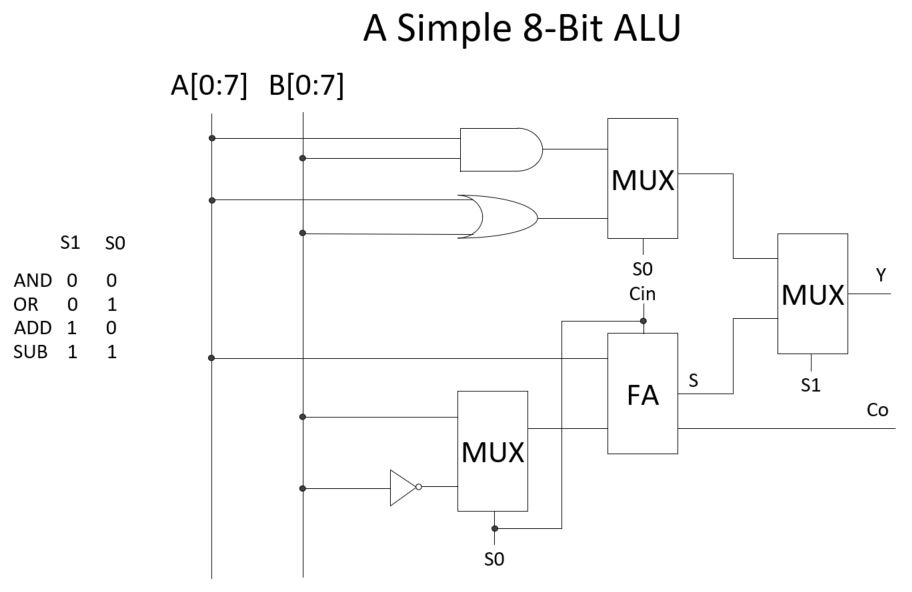Next, build the schematic in Electric. (You need the 8-bit version of each module shown in the diagram above). For example I am missing the 8-bit inverter in my library.The schematic of the 8-bit ALU: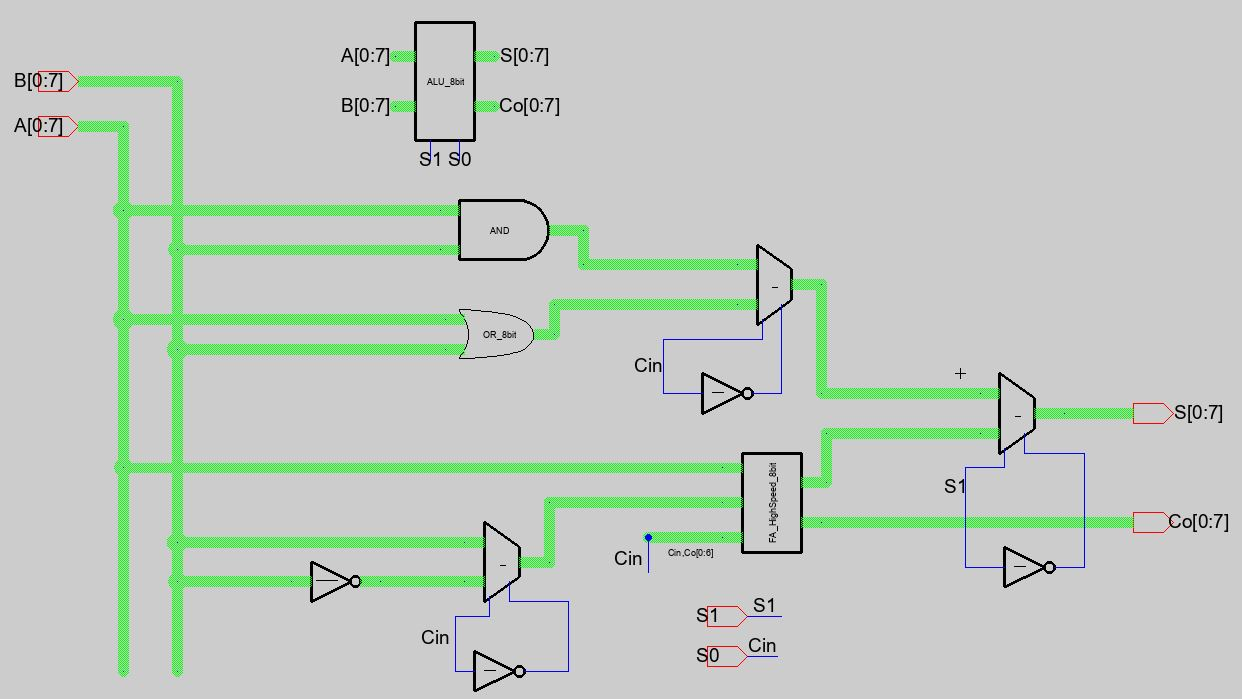Next, run the simulation:

First, verify the AND gate by setting S1S0 = 00:

1111 1111 AND 0000 0000 should be all 0's.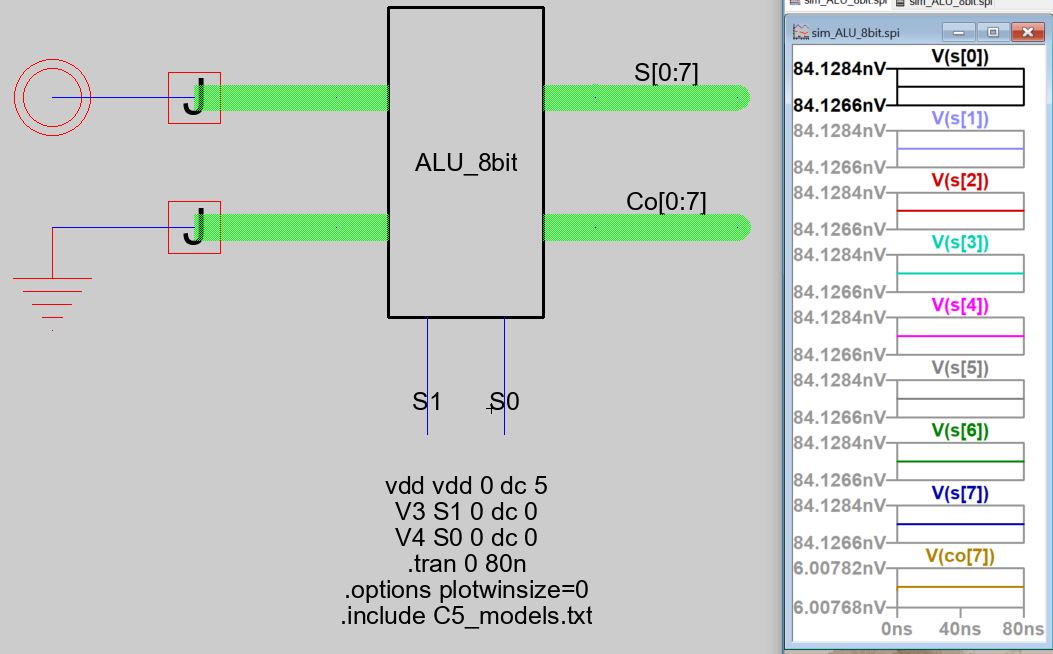Second, verify the OR gate by setting S1S0 = 01:

1111 1111 OR 0000 0000 should be all 1's.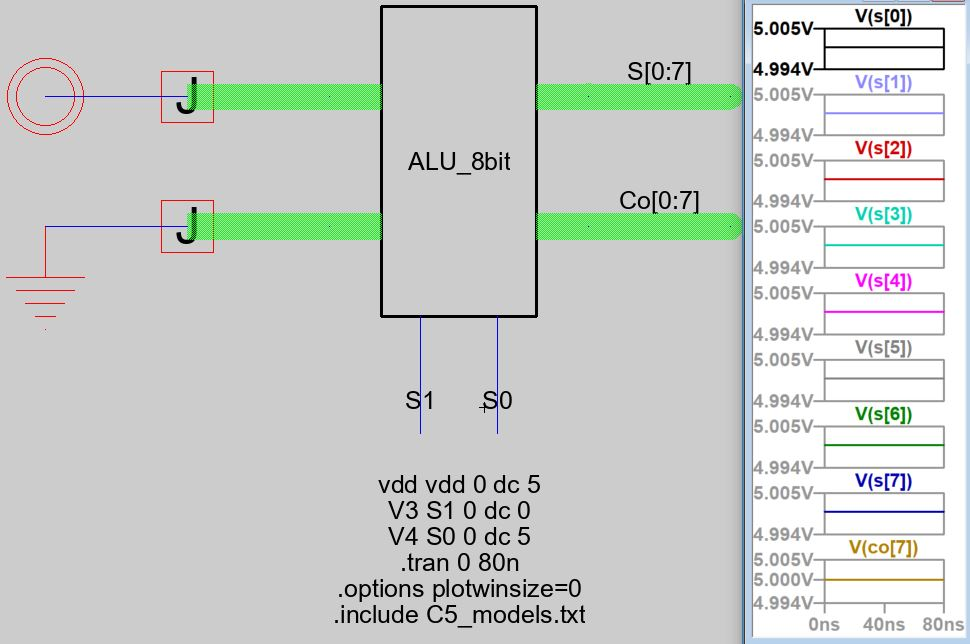Next, verify the ADD operation. Use 1111 1111 + 0000 0001 = S = 0000 0000, Co = 1.Next, verify the subtraction operation. Use 1111 1111 - 0000 0001 = 1111 1111 + (-0000 0001) = 1111 1111 + (1111 1110 + 1) = 1111 1111 + 1111 1111 = 1111 1110 (Co = 1).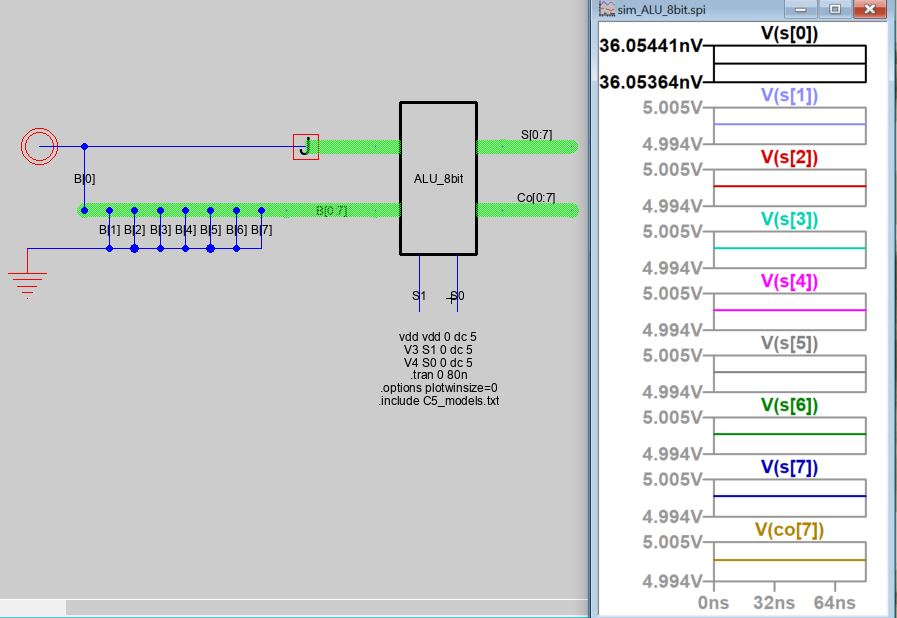Task 2: Finish the layout of the ALU. (50 points)

Watch this short video for the tricks of wiring a large scale integrated circuit by hand. The rule of thumb is for wiring in between two modules, keep the traces from the same metal layer consistently horizontal or vertical.

The finished layout looks like this: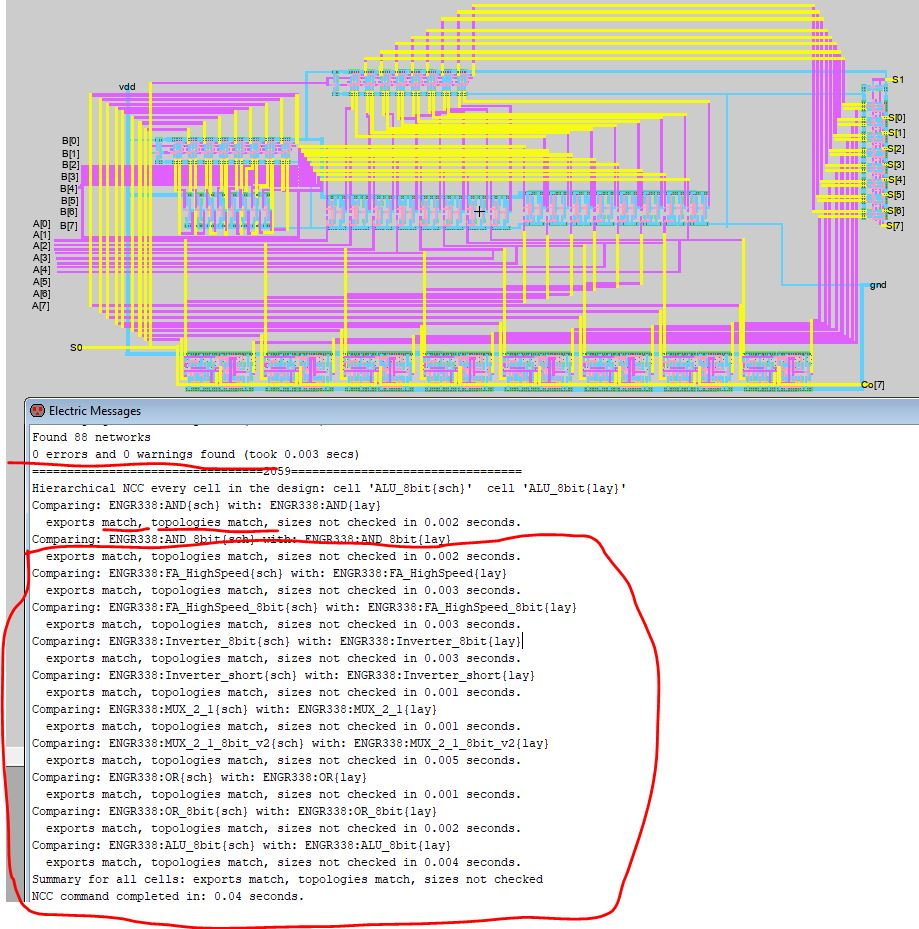I don't need to report all the Co[0:7] ports so I went back to the schematic to only export Co. Don't forget to make the same changes to any subcells that are being affected by this change. (like the simulation one view).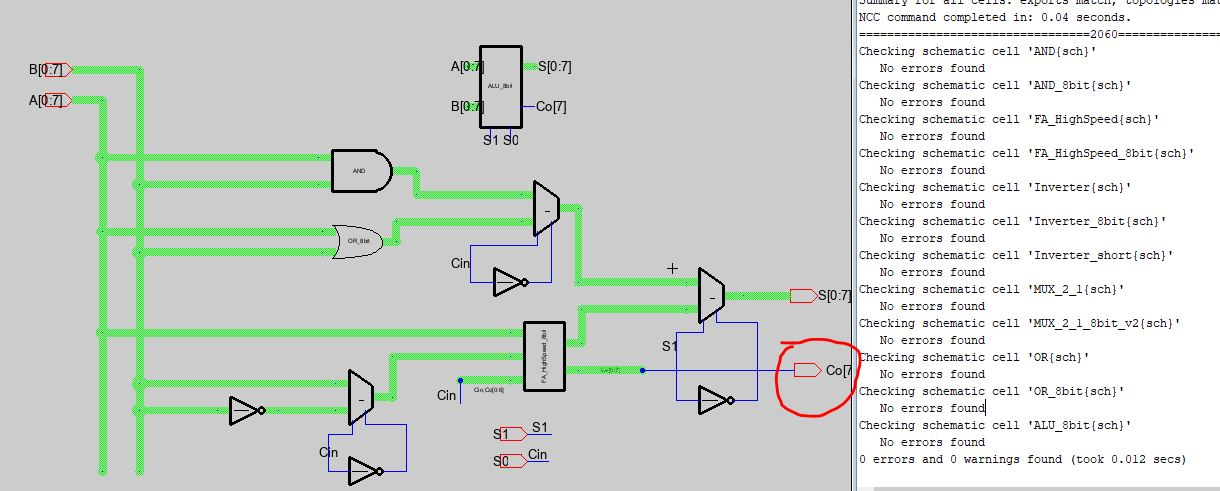An bigger image of the layout can be seen below (click the figure to zoom in). One thing to let you know is at some point, you should start designing this on your own instead of following what I have in the example. Your layout and location of the ports canbe totally different. If you just follow mine, you may run into errors and troubles.
Start think about the topology and the design by yourself starting from the schematic in Task 1.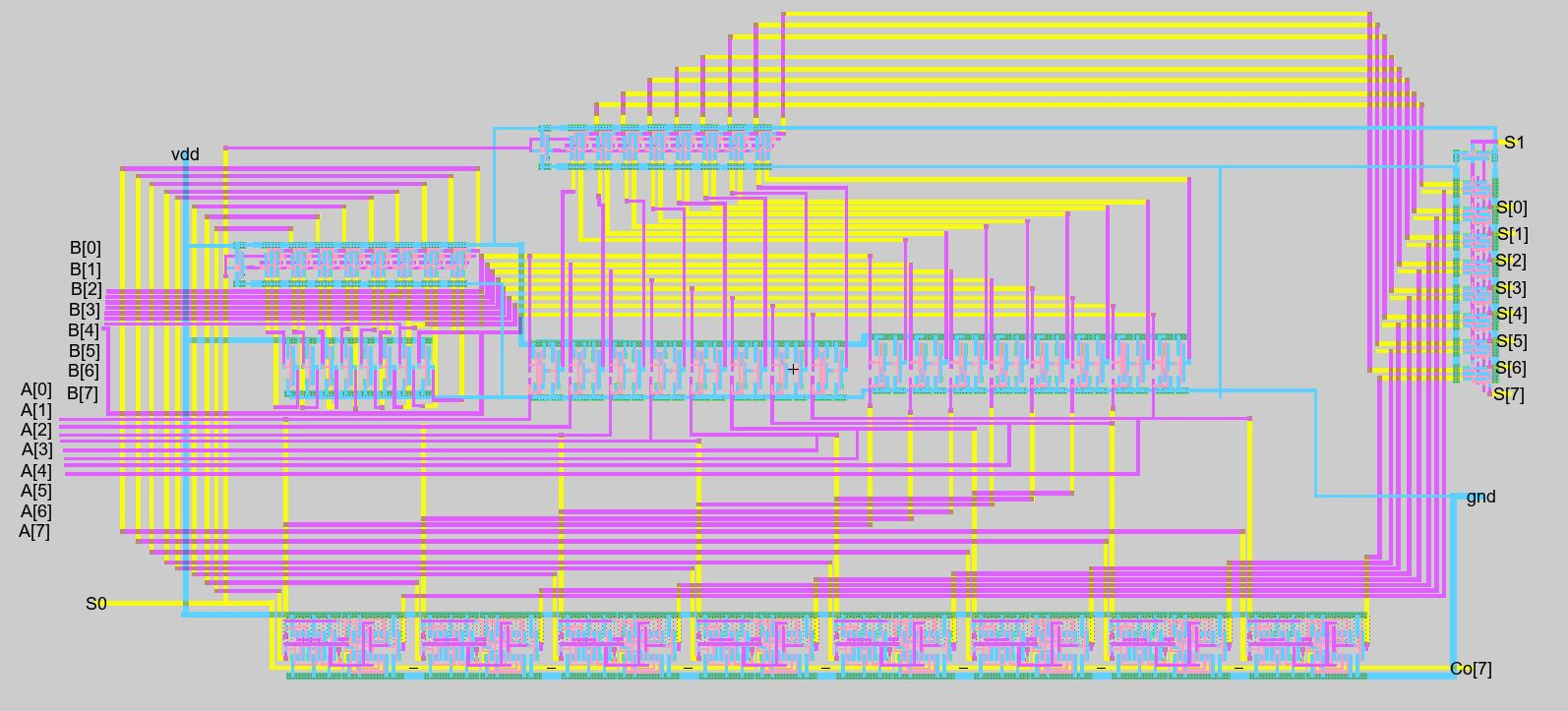The one with labels: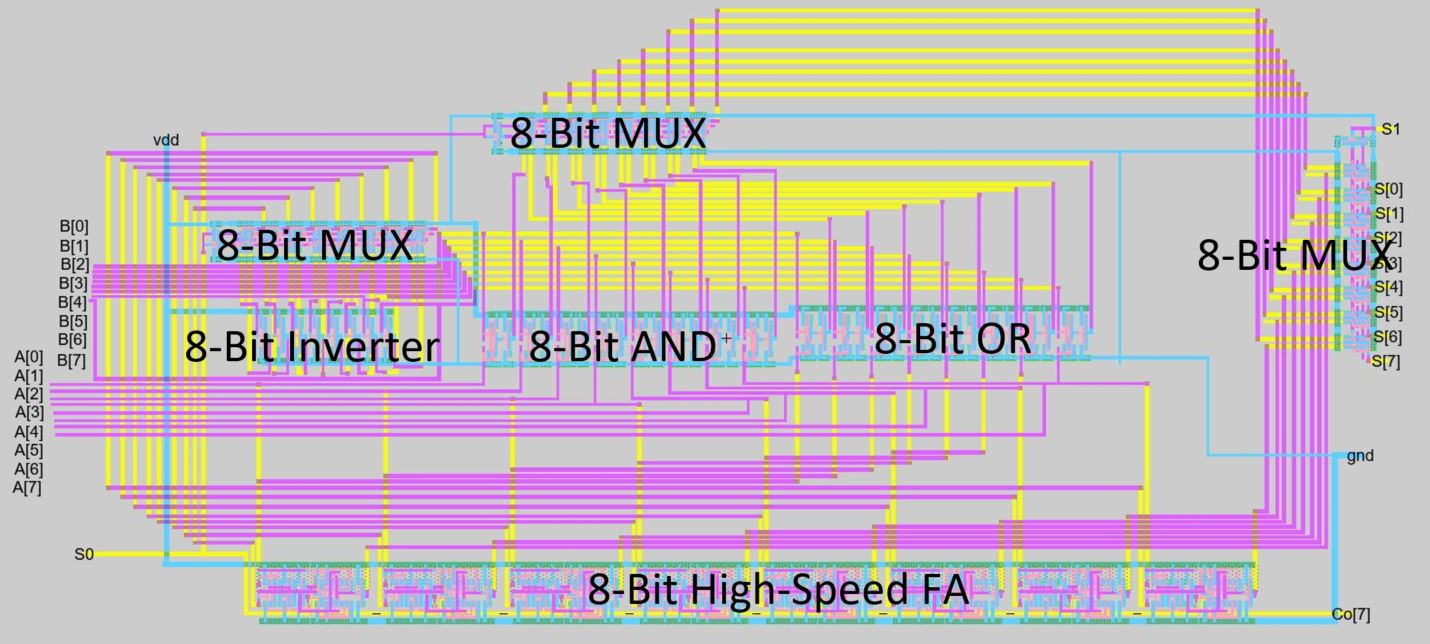Lab reports grading rubric:

Task 1: 40 points.
Task 2: 50 points.
Writing/formatting: 10 points.

---------------------------------This is the end of the lab